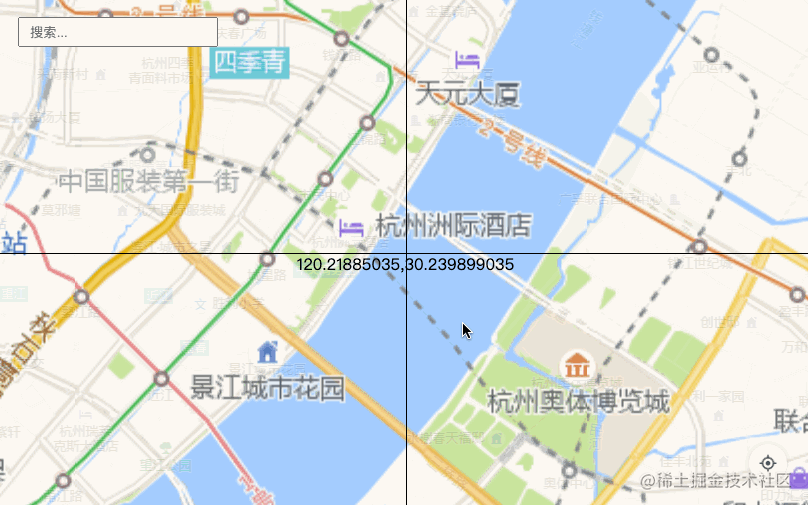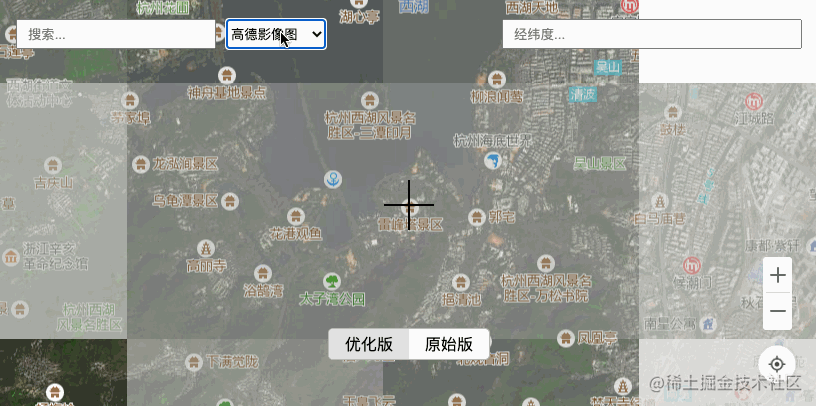# 从零打造一个Web地图引擎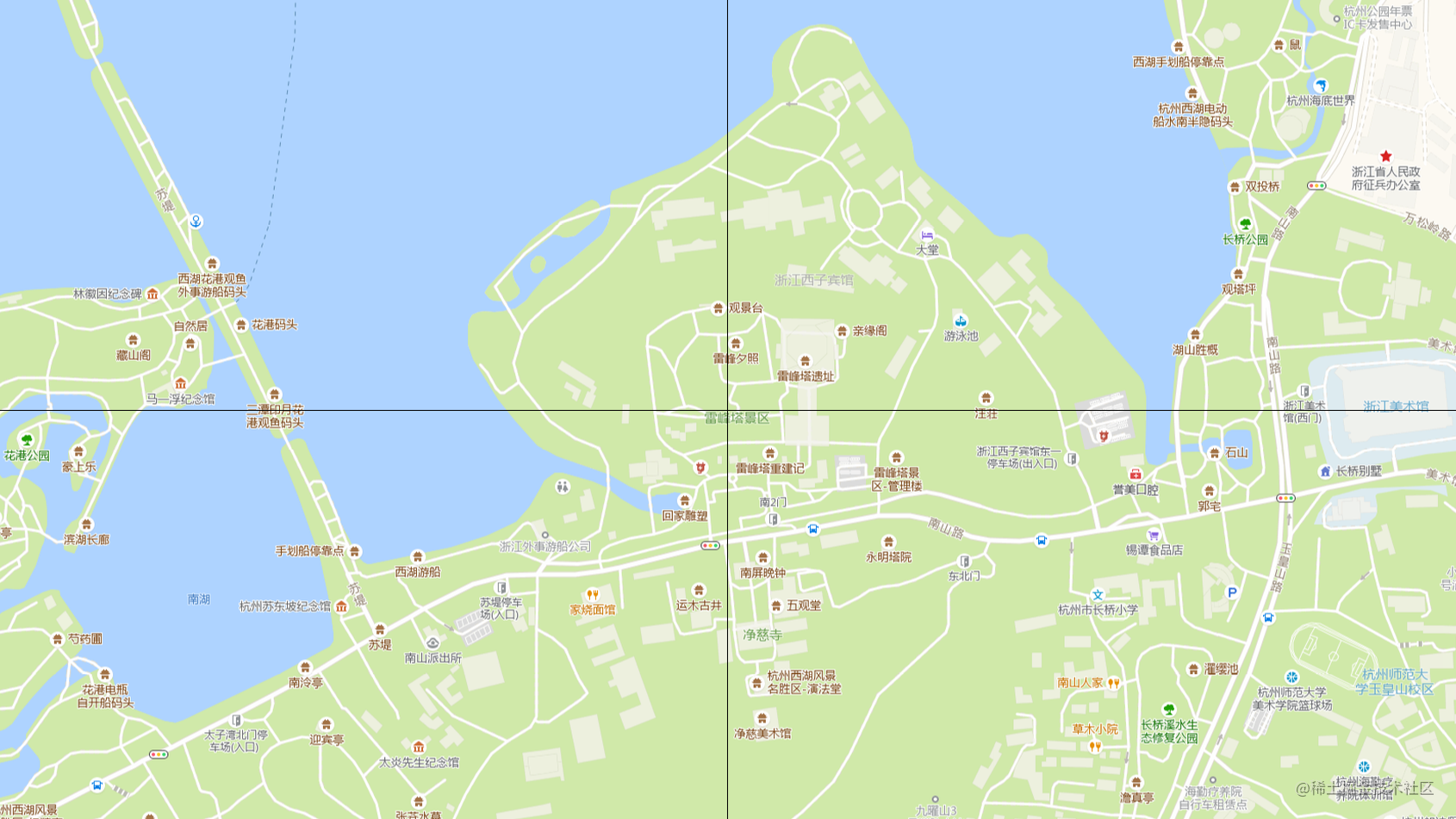小小小小前端 @ 你猜

# 选个经纬度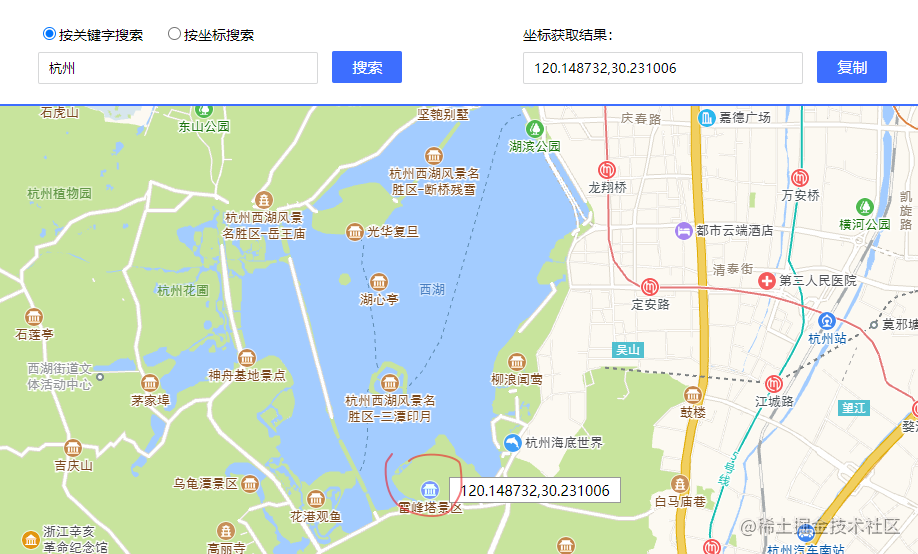# 瓦片url分析

``````https://webrd0{1-4}.is.autonavi.com/appmaptile?x={x}&y={y}&z={z}&lang=zh_cn&size=1&scale=1&style=8

TMS规范：腾讯地图，坐标原点在左下角

WMTS规范：原点在左上角，瓦片不是正方形，而是矩形，这个应该是官方标准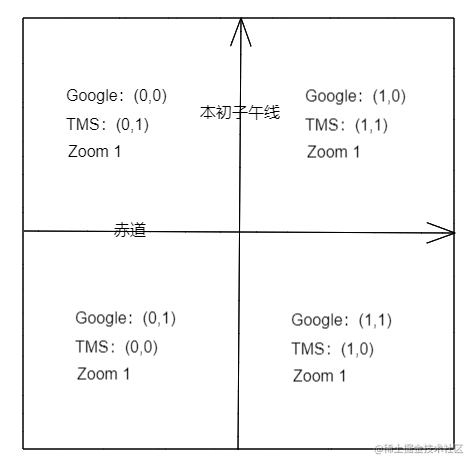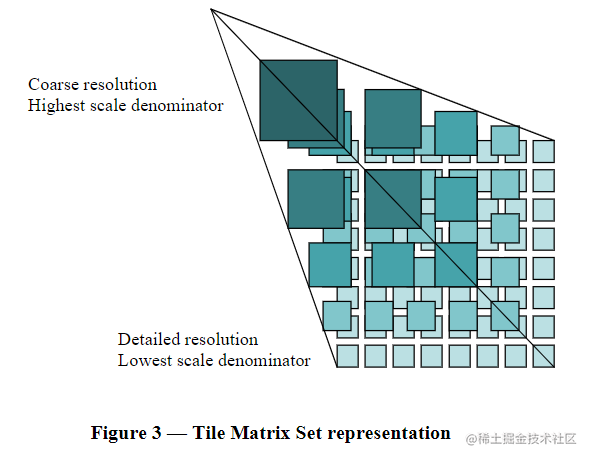``````Math.pow(Math.pow(2, n), 2)// 行*列：2^n * 2^n

``````x：行号
y：列号
z：分辨率，一般为0-18

``````https://webrd01.is.autonavi.com/appmaptile?x=109280&y=53979&z=17&lang=zh_cn&size=1&scale=1&style=8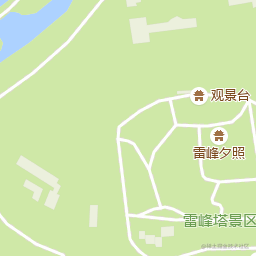# 坐标系简介

`WGS-84`坐标系是国际通用的标准，`EPSG`编号为`EPSG:4326`，通常GPS设备获取到的原始经纬度和国外的地图厂商使用的都是`WGS-84`坐标系。

# 经纬度定位行列号

``````// 角度转弧度
const angleToRad = (angle) => {
return angle * (Math.PI / 180)
}

// 弧度转角度
return rad * (180 / Math.PI)
}

// 地球半径

// 4326转3857
const lngLat2Mercator = (lng, lat) => {
// 经度先转弧度，然后因为 弧度 = 弧长 / 半径 ，得到弧长为 弧长 = 弧度 * 半径
// 纬度先转弧度
// 下面我就看不懂了，各位随意。。。
let y = EARTH_RAD / 2 * Math.log((1 + sin) / (1 - sin))
return [x, y]
}

// 3857转4326
const mercatorTolnglat = (x, y) => {
let lat = radToAngle((2 * Math.atan(Math.exp(y / EARTH_RAD)) - (Math.PI / 2)))
return [lng, lat]
}

`3857`坐标有了，它的单位是`米`，那么怎么转换成瓦片的行列号呢，这就涉及到`分辨率`的概念了，即地图上一像素代表实际多少米，分辨率如果能从地图厂商的文档里获取是最好的，如果找不到，也可以简单计算一下（如果使用计算出来的也不行，那就只能求助搜索引擎了），我们知道地球半径是`6378137`米，`3857`坐标系把地球当做正圆球体来处理，所以可以算出地球周长，投影是贴着地球赤道的：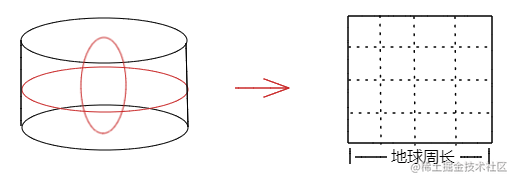``````// 地球周长
const EARTH_PERIMETER = 2 * Math.PI * EARTH_RAD
// 瓦片像素
const TILE_SIZE = 256

// 获取某一层级下的分辨率
const getResolution = (n) => {
const tileNums = Math.pow(2, n)
const tileTotalPx = tileNums * TILE_SIZE
return EARTH_PERIMETER / tileTotalPx
}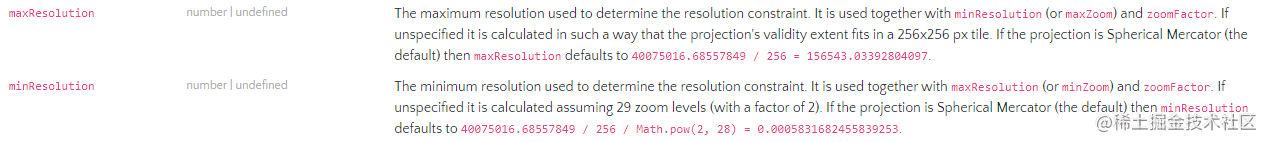`3857`坐标的单位是`米`，那么把坐标除以分辨率就可以得到对应的像素坐标，再除以`256`，就可以得到瓦片的行列号：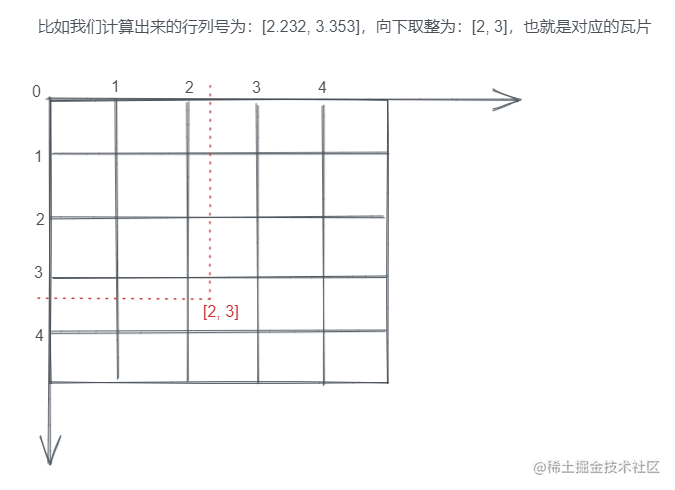``````// 根据3857坐标及缩放层级计算瓦片行列号
const getTileRowAndCol = (x, y, z) => {
let resolution = getResolution(z)
let row = Math.floor(x / resolution / TILE_SIZE)
let col = Math.floor(y / resolution / TILE_SIZE)
return [row, col]
}

``````https://webrd01.is.autonavi.com/appmaptile?x=43744&y=11556&z=17&lang=zh_cn&size=1&scale=1&style=8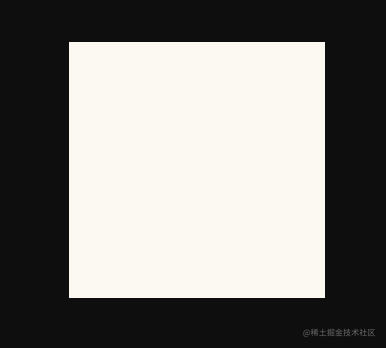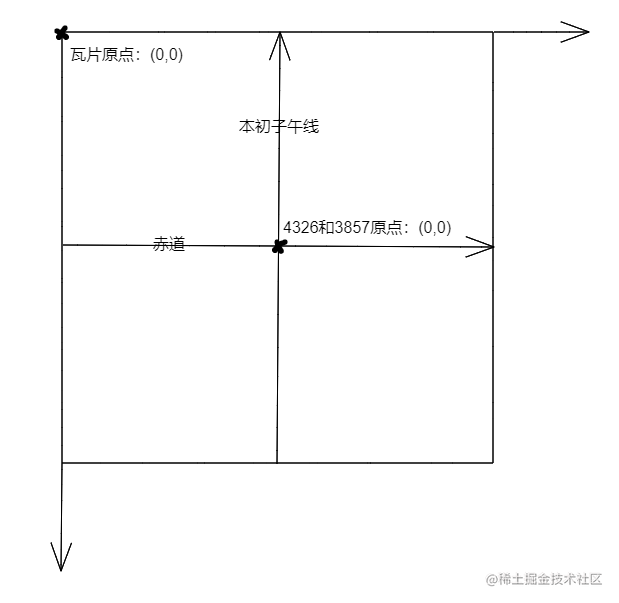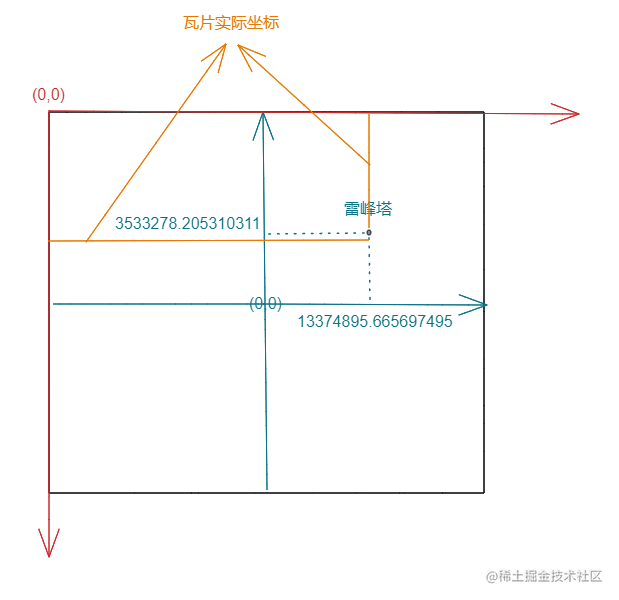`3857`坐标系的原点相当于在世界平面图的中间，向右为`x`轴正方向，向上为`y`轴正方向，而瓦片地图的原点在左上角，所以我们需要根据图上【绿色虚线】的距离计算出【橙色实线】的距离，这也很简单，水平坐标就是水平绿色虚线的长度加上世界平面图的一半，垂直坐标就是世界平面图的一半减去垂直绿色虚线的长度，世界平面图的一半也就是地球周长的一半，修改`getTileRowAndCol`函数：

``````const getTileRowAndCol = (x, y, z) => {
x += EARTH_PERIMETER / 2     // ++
y = EARTH_PERIMETER / 2 - y  // ++
let resolution = getResolution(z)
let row = Math.floor(x / resolution / TILE_SIZE)
let col = Math.floor(y / resolution / TILE_SIZE)
return [row, col]
}

``````https://webrd01.is.autonavi.com/appmaptile?x=109280&y=53979&z=17&lang=zh_cn&size=1&scale=1&style=8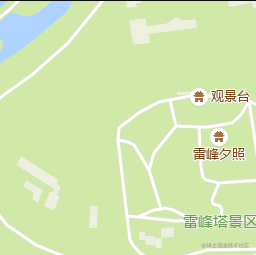# 瓦片显示位置计算

``````<template>
<div class="map" ref="map">
<canvas ref="canvas"></canvas>
</div>
</template>

``````// 容器大小
let { width, height } = this.\$refs.map.getBoundingClientRect()
this.width = width
this.height = height
// 设置画布大小
let canvas = this.\$refs.canvas
canvas.width = width
canvas.height = height
// 获取绘图上下文
this.ctx = canvas.getContext('2d')

``````// 中心点对应的瓦片
let centerTile = getTileRowAndCol(
...lngLat2Mercator(...this.center),// 4326转3857
this.zoom// 缩放层级
)

``````// 中心瓦片左上角对应的像素坐标
let centerTilePos = [centerTile * TILE_SIZE, centerTile * TILE_SIZE]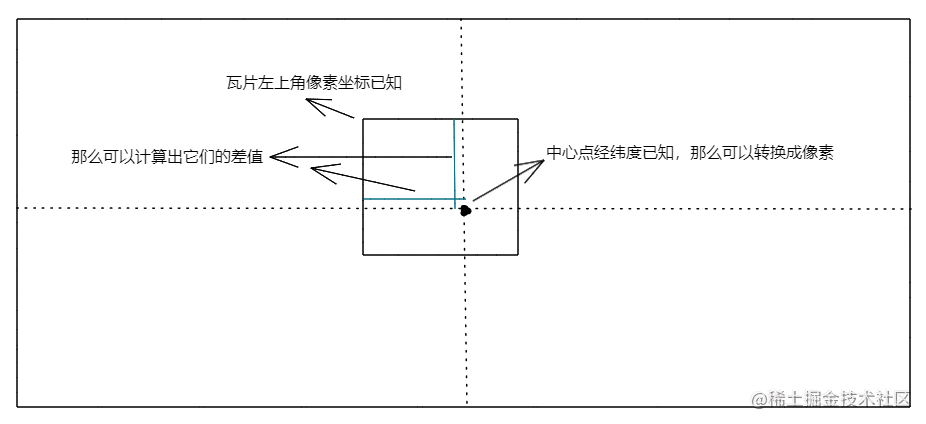``````// 计算4326经纬度对应的像素坐标
const getPxFromLngLat = (lng, lat, z) => {
let [_x, _y] = lngLat2Mercator(lng, lat)// 4326转3857
// 转成世界平面图的坐标
_x += EARTH_PERIMETER / 2
_y = EARTH_PERIMETER / 2 - _y
let resolution = resolutions[z]// 该层级的分辨率
// 米/分辨率得到像素
let x = Math.floor(_x / resolution)
let y = Math.floor(_y / resolution)
return [x, y]
}

``````// 中心点对应的像素坐标
let centerPos = getPxFromLngLat(...this.center, this.zoom)

``````// 中心像素坐标距中心瓦片左上角的差值
let offset = [
centerPos - centerTilePos,
centerPos - centerTilePos
]

``````// 移动画布原点到画布中间
this.ctx.translate(this.width / 2, this.height / 2)
// 加载瓦片图片
let img = new Image()
// 拼接瓦片地址
img.src = getTileUrl(...centerTile, this.zoom)
// 渲染到canvas
this.ctx.drawImage(img, -offset, -offset)
}

``````// 拼接瓦片地址
const getTileUrl = (x, y, z) => {
let domainIndexList = [1, 2, 3, 4]
let domainIndex =
domainIndexList[Math.floor(Math.random() * domainIndexList.length)]
return `https://webrd0\${domainIndex}.is.autonavi.com/appmaptile?x=\${x}&y=\${y}&z=\${z}&lang=zh_cn&size=1&scale=1&style=8`
}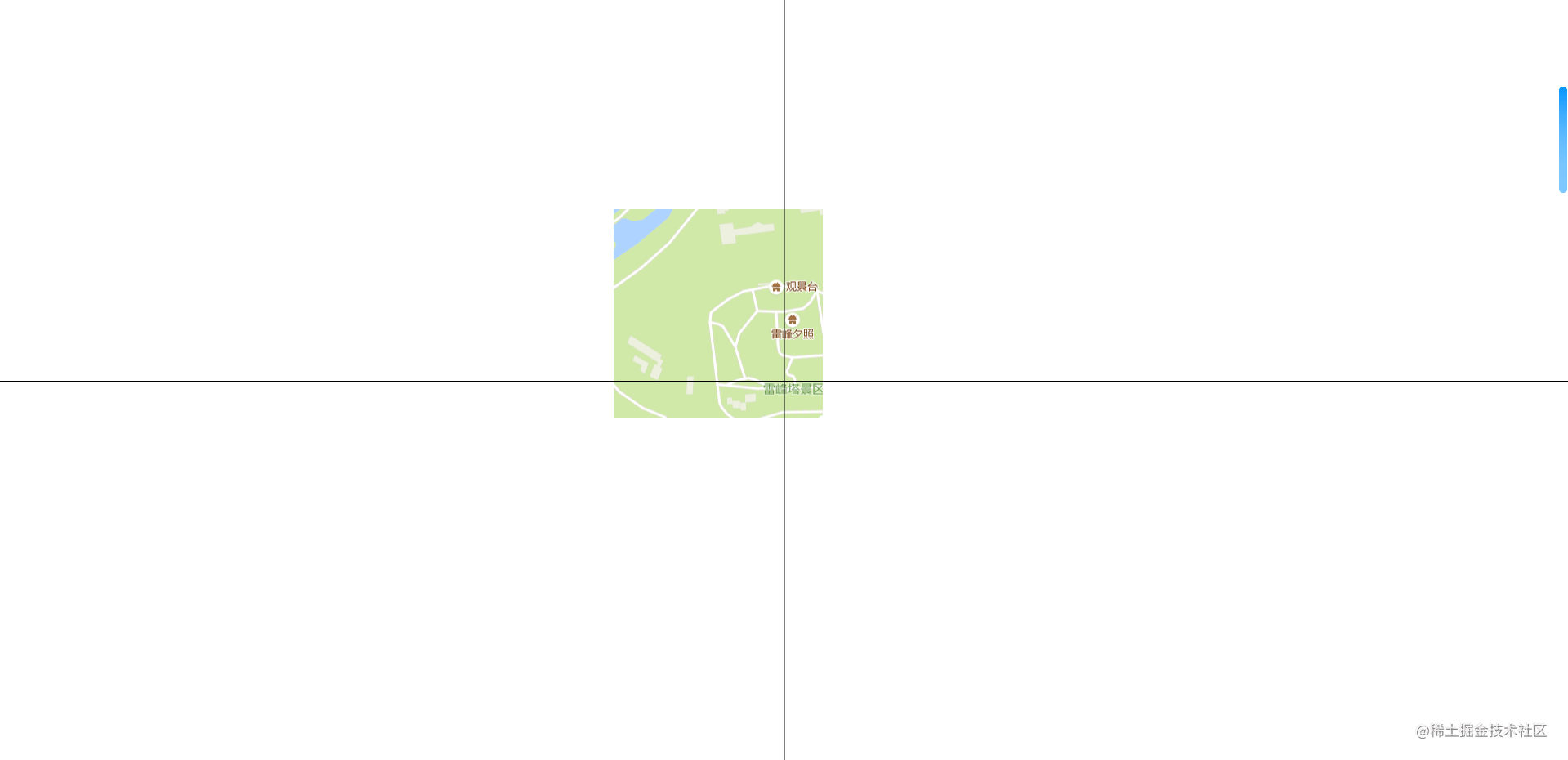``````// 瓦片行列号，行号减1，列号不变
let leftTile = [centerTile - 1, centerTile]
// 瓦片显示坐标，x轴减去一个瓦片的大小，y轴不变
let leftTilePos = [
offset - TILE_SIZE * 1,
offset
]``````// 计算瓦片数量
let rowMinNum = Math.ceil((this.width / 2 - offset) / TILE_SIZE)// 左
let colMinNum = Math.ceil((this.height / 2 - offset) / TILE_SIZE)// 上
let rowMaxNum = Math.ceil((this.width / 2 - (TILE_SIZE - offset)) / TILE_SIZE)// 右
let colMaxNum = Math.ceil((this.height / 2 - (TILE_SIZE - offset)) / TILE_SIZE)// 下

``````// 从上到下，从左到右，加载瓦片
for (let i = -rowMinNum; i <= rowMaxNum; i++) {
for (let j = -colMinNum; j <= colMaxNum; j++) {
// 加载瓦片图片
let img = new Image()
img.src = getTileUrl(
centerTile + i,// 行号
centerTile + j,// 列号
this.zoom
)
// 渲染到canvas
this.ctx.drawImage(
img,
i * TILE_SIZE - offset,
j * TILE_SIZE - offset
)
}
}
}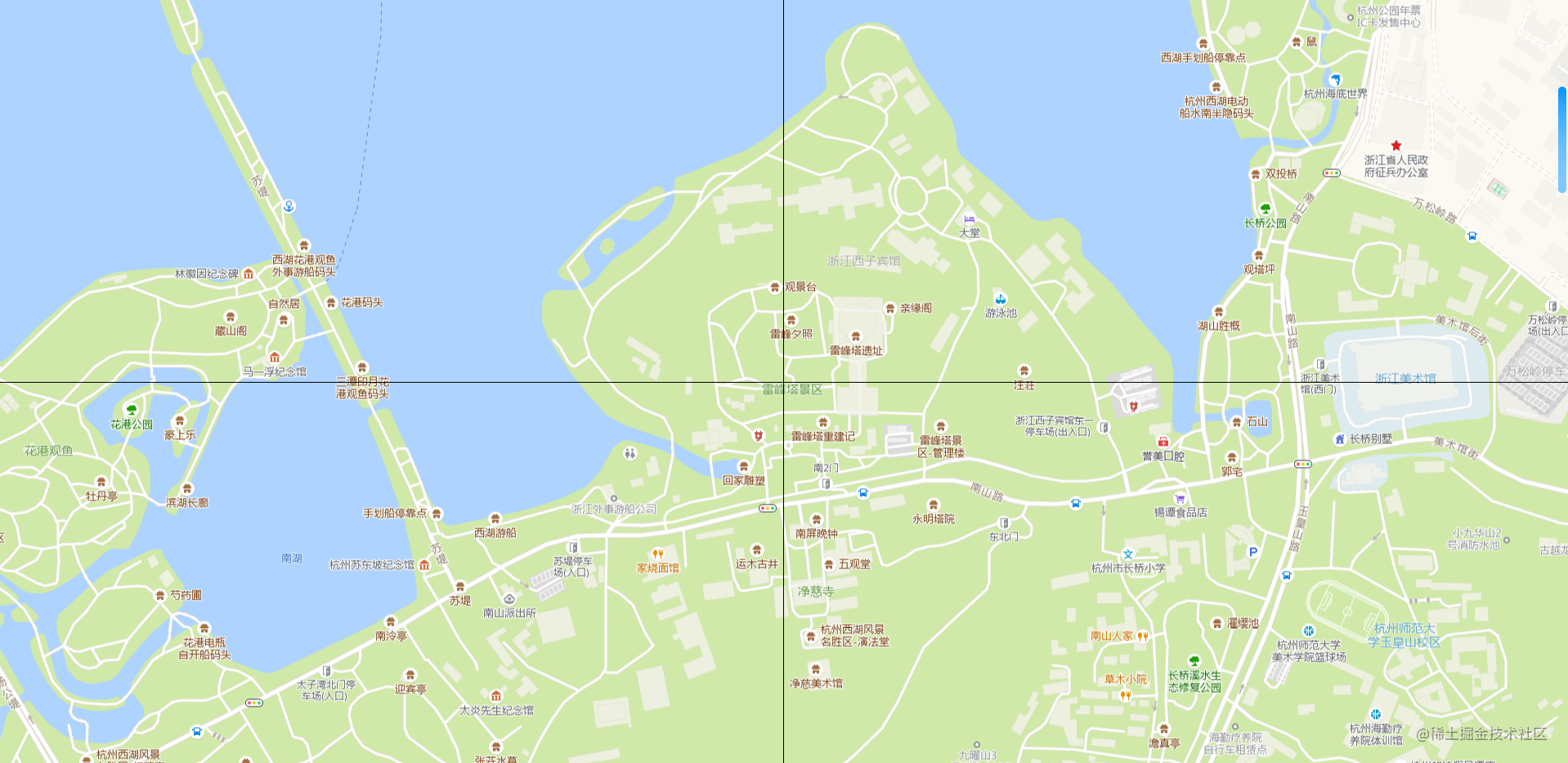# 拖动

``````<canvas ref="canvas" @mousedown="onMousedown"></canvas>

``````export default {
data(){
return {
isMousedown: false
}
},
mounted() {
},
methods: {
// 鼠标按下
onMousedown(e) {
if (e.which === 1) {
this.isMousedown = true;
}
},

// 鼠标移动
onMousemove(e) {
if (!this.isMousedown) {
return;
}
// ...
},

// 鼠标松开
onMouseup() {
this.isMousedown = false;
}
}
}

`onMousemove`方法里计算拖动后的中心经纬度及重新渲染画布：

``````// 计算本次拖动的距离对应的经纬度数据
let mx = e.movementX * resolutions[this.zoom];
let my = e.movementY * resolutions[this.zoom];
// 把当前中心点经纬度转成3857坐标
let [x, y] = lngLat2Mercator(...this.center);
// 更新拖动后的中心点经纬度
center = mercatorToLngLat(x - mx, my + y);

`movementX``movementY`属性能获取本次和上一次鼠标事件中的移动值，兼容性不是很好，不过自己计算该值也很简单，详细请移步MDN。乘以当前分辨率把`像素`换算成`米`，然后把当前中心点经纬度也转成`3857``米`坐标，偏移本次移动的距离，最后再转回`4326`的经纬度坐标作为更新后的中心点即可。

``````// 清空画布
this.clear();
// 重新绘制，renderTiles方法就是上一节的代码逻辑封装
this.renderTiles();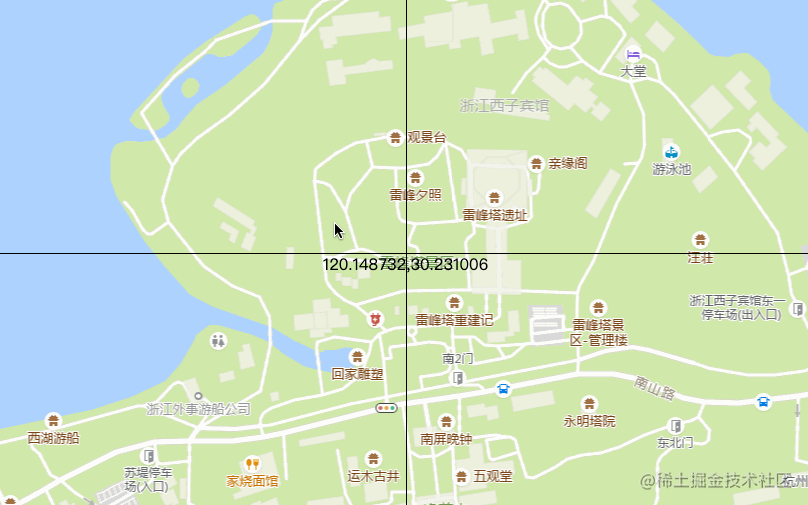``````{
// 缓存瓦片
tileCache: {},
// 记录当前画布上需要的瓦片
currentTileCache: {}
}

``````// 瓦片类
class Tile {
constructor(opt = {}) {
// 画布上下文
this.ctx = ctx
// 瓦片行列号
this.row = row
this.col = col
// 瓦片层级
this.zoom = zoom
// 显示位置
this.x = x
this.y = y
// 一个函数，判断某块瓦片是否应该渲染
this.shouldRender = shouldRender
// 瓦片url
this.url = ''
// 缓存key
this.cacheKey = this.row + '_' + this.col + '_' + this.zoom
// 图片
this.img = null
// 图片是否加载完成

this.createUrl()
}

// 生成url
createUrl() {
this.url = getTileUrl(this.row, this.col, this.zoom)
}

// 加载图片
this.img = new Image()
this.img.src = this.url
this.render()
}
}

// 将图片渲染到canvas上
render() {
return
}
this.ctx.drawImage(this.img, this.x, this.y)
}

// 更新位置
updatePos(x, y) {
this.x = x
this.y = y
return this
}
}

``````this.currentTileCache = {}// 清空缓存对象
for (let i = -rowMinNum; i <= rowMaxNum; i++) {
for (let j = -colMinNum; j <= colMaxNum; j++) {
// 当前瓦片的行列号
let row = centerTile + i
let col = centerTile + j
// 当前瓦片的显示位置
let x = i * TILE_SIZE - offset
let y = j * TILE_SIZE - offset
// 缓存key
let cacheKey = row + '_' + col + '_' + this.zoom
// 记录画布当前需要的瓦片
this.currentTileCache[cacheKey] = true
// 该瓦片已加载过
if (this.tileCache[cacheKey]) {
// 更新到当前位置
this.tileCache[cacheKey].updatePos(x, y).render()
} else {
// 未加载过
this.tileCache[cacheKey] = new Tile({
ctx: this.ctx,
row,
col,
zoom: this.zoom,
x,
y,
// 判断瓦片是否在当前画布缓存对象上，是的话则代表需要渲染
shouldRender: (key) => {
return this.currentTileCache[key]
},
})
}
}
}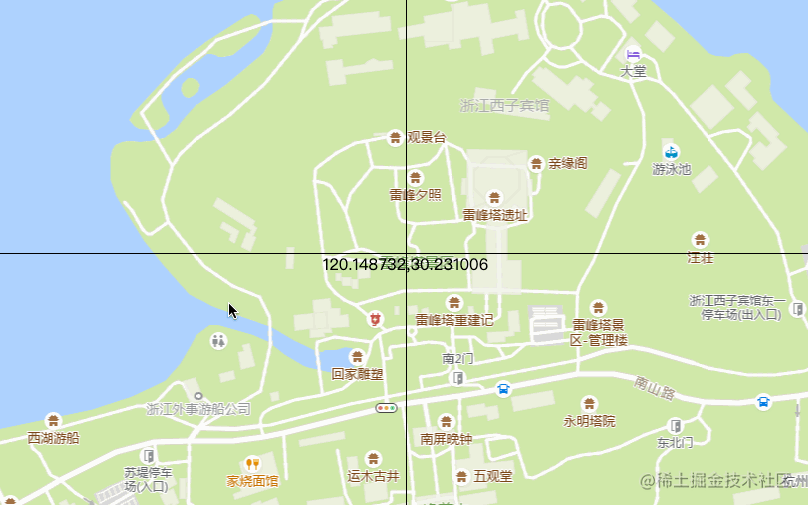1.一般会先排个序，优先加载中心瓦片

2.缓存的瓦片越来越多肯定也会影响性能，所以还需要一些清除策略

# 缩放

``````export default {
data() {
return {
// 缩放层级范围
minZoom: 3,
maxZoom: 18,
// 防抖定时器
zoomTimer: null
}
},
mounted() {
},
methods: {
// 鼠标滚动
onMousewheel(e) {
if (e.deltaY > 0) {
// 层级变小
if (this.zoom > this.minZoom) this.zoom--
} else {
// 层级变大
if (this.zoom < this.maxZoom) this.zoom++
}
// 加个防抖，防止快速滚动加载中间过程的瓦片
this.zoomTimer = setTimeout(() => {
this.clear()
this.renderTiles()
}, 300)
}
}
}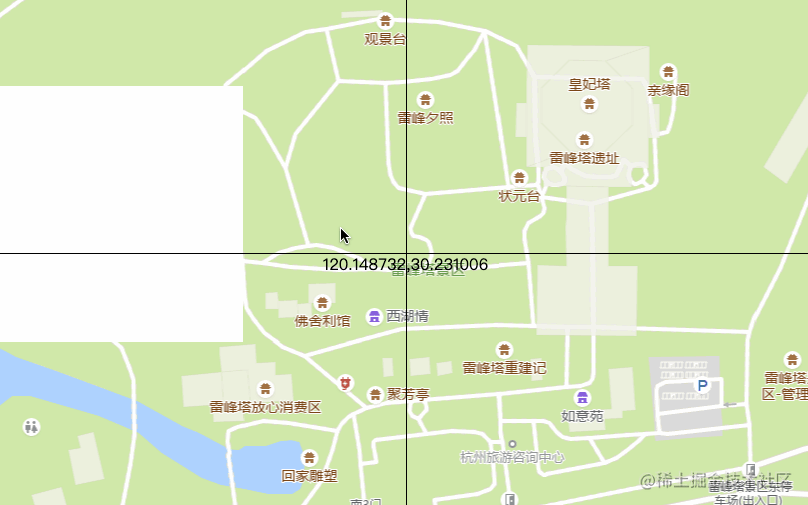``````// 动画使用popmotion库，https://popmotion.io/
import { animate } from 'popmotion'

export default {
data() {
return {
lastZoom: 0,
scale: 1,
scaleTmp: 1,
playback: null,
}
},
methods: {
// 鼠标滚动
onMousewheel(e) {
if (e.deltaY > 0) {
// 层级变小
if (this.zoom > this.minZoom) this.zoom--
} else {
// 层级变大
if (this.zoom < this.maxZoom) this.zoom++
}
// 层级未发生改变
if (this.lastZoom === this.zoom) {
return
}
this.lastZoom = this.zoom
// 更新缩放比例，也就是目标缩放值
this.scale *= e.deltaY > 0 ? 0.5 : 2
// 停止上一次动画
if (this.playback) {
this.playback.stop()
}
// 开启动画
this.playback = animate({
from: this.scaleTmp,// 当前缩放值
to: this.scale,// 目标缩放值
onUpdate: (latest) => {
// 实时更新当前缩放值
this.scaleTmp = latest
// 保存画布之前状态，原因有二：
// 1.scale方法是会在之前的状态上叠加的，比如初始是1，第一次执行scale(2,2)，第二次执行scale(3,3)，最终缩放值不是3，而是6，所以每次缩放完就恢复状态，那么就相当于每次都是从初始值1开始缩放，效果就对了
// 2.保证缩放效果只对重新渲染已有瓦片生效，不会对最后的renderTiles()造成影响
this.ctx.save()
this.clear()
this.ctx.scale(latest, latest)
// 刷新当前画布上的瓦片
Object.keys(this.currentTileCache).forEach((tile) => {
this.tileCache[tile].render()
})
// 恢复到画布之前状态
this.ctx.restore()
},
onComplete: () => {
// 动画完成后将缩放值重置为1
this.scale = 1
this.scaleTmp = 1
// 根据最终缩放值重新计算需要的瓦片并渲染
this.renderTiles()
},
})
}
}
}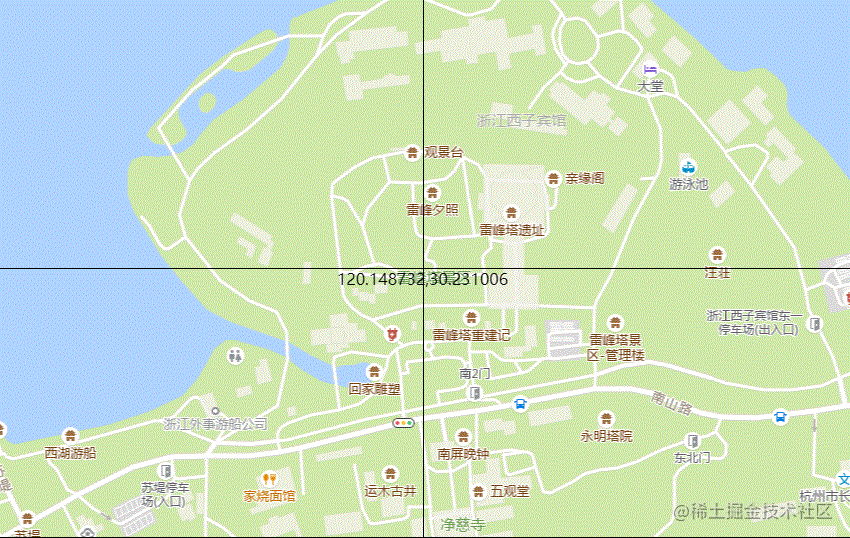# 总结